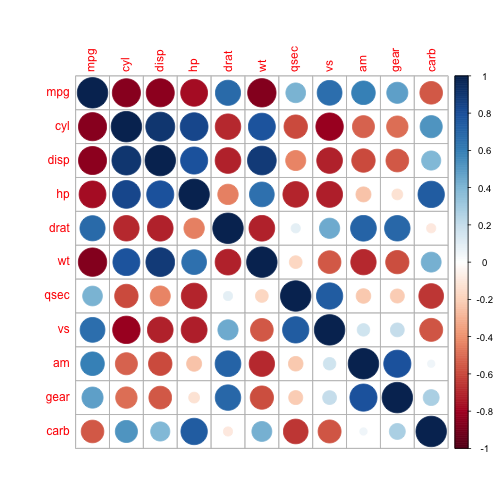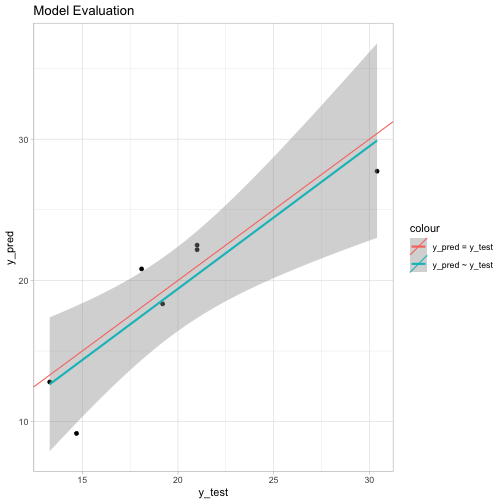# Introduction to R Plumber : Expose a Caret model to a web API

In this post we explore the basics of the Plumber package. Our aim is to ilustrate how to fit a $$L^2$$-regularized linear model and expose it to a web API so that we can request predictions.

## Prepare Notebook

Let us load the necessary libraries.

library(caret)
library(httr)
library(magrittr)
library(plumber)
library(tidyverse)

As a toy example we consider the mtcars data set.

df <- mtcars %>% as_tibble()

df %>% head
## # A tibble: 6 x 11
##     mpg   cyl  disp    hp  drat    wt  qsec    vs    am  gear  carb
##   <dbl> <dbl> <dbl> <dbl> <dbl> <dbl> <dbl> <dbl> <dbl> <dbl> <dbl>
## 1  21       6   160   110  3.9   2.62  16.5     0     1     4     4
## 2  21       6   160   110  3.9   2.88  17.0     0     1     4     4
## 3  22.8     4   108    93  3.85  2.32  18.6     1     1     4     1
## 4  21.4     6   258   110  3.08  3.22  19.4     1     0     3     1
## 5  18.7     8   360   175  3.15  3.44  17.0     0     0     3     2
## 6  18.1     6   225   105  2.76  3.46  20.2     1     0     3     1

We want to fit a simple linear model to predict the variable mpg.

Warning: We are not interested to find the “best model”. Better feature engineering and hyperparameter tunig is not developed here because it is not the main purpose.

## Correlation Plot

For variable selection we consider a correlation plot.

df %>% cor %>% corrplot::corrplot()From the visualization we see that the variables wt, qsec and am could be good predictors.

## Define and Train Model

We are going to use the Caret package.

### Split Data

set.seed(seed = 0)

# Define observation matrix.
X <- df %>% select(wt, qsec, am)
# Define target vector.
y <- df %>% pull(mpg)

# Define a partition of the data.
partition <- createDataPartition(y = y, p = 0.75, list = FALSE)

# Split the data into a training and test set.
df.train <- df[partition, ]
df.test <- df[- partition, ]

X.train <- df.train %>% select(wt, qsec, am)
y.train <- df.train %>% pull(mpg)

X.test <- df.test %>% select(wt, qsec, am)
y.test <- df.test %>% pull(mpg)

### Data Pre-Processing

As we want to use a linear model, we neet to scale the variables.

# Define scaler object.
scaler.obj <- preProcess(x = X.train, method = c('center', 'scale'))

# Transform the data.
X.train.scaled <- predict(object = scaler.obj, newdata = X.train)
X.test.scaled <- predict(object = scaler.obj, newdata = X.test)

### Train Model

We fit $$L^2$$-regularization linear model using a 3-fold cross-validation to tune the regularization hyperparameter.

model.obj <-  train(x = X.train.scaled,
y = y.train,
method = 'ridge',
trControl = trainControl(method = 'cv', number = 3),
metric = 'RMSE')

### Model Evaluation

Let us evaluate the model perforance.

On the training set:

model.obj$results %>% select(RMSE) ## RMSE ## 1 2.613844 ## 2 2.613700 ## 3 2.554912 On the test set: y.pred <- predict(model.obj, newdata = X.test.scaled) RMSE(pred = y.pred, obs = y.test) ##  2.664047 The model seems to be stable and there is no strong evidence of overfitting. ### Visualization tibble(y_test = y.test, y_pred = y.pred) %>% ggplot() + theme_light() + geom_point(mapping = aes(x = y_test, y = y_pred)) + geom_smooth(mapping = aes(x = y_test, y = y_pred, colour = 'y_pred ~ y_test'), method = 'lm', formula = y ~ x) + geom_abline(mapping = aes(slope = 1, intercept = 0, colour = 'y_pred = y_test'), show.legend = TRUE) + ggtitle(label = 'Model Evaluation')## Save Model ### Data Pipeline We define a function which transforms and predicts for new incoming data. GetNewPredictions <- function(model, transformer, newdata){ newdata.transformed <- predict(object = transformer, newdata = newdata) new.predictions <- predict(object = model, newdata = newdata.transformed) return(new.predictions) } ### Save Output Object # Define Output object. model.list <- vector(mode = 'list') # Save scaler object. model.list$scaler.obj <- scaler.obj
# Save fitted model.
model.list$model.obj <- model.obj # Save transformation function. model.list$GetNewPredictions <- GetNewPredictions

saveRDS(object = model.list, file = 'model_list.rds')

## Write Plumber Script

This is the basic structure of a Plumber script.

# plumber.R

#* @param wt
#* @param qsec
#* @param am
#* @post /predict
CalculatePrediction <- function(wt, qsec, am){

wt %<>% as.numeric
qsec %<>% as.numeric
am %<>% as.numeric

X.new <- tibble(wt = wt, qsec = qsec, am = am)

y.pred <- model.list$GetNewPredictions(model = model.list$model.obj,
transformer = model.list$scaler.obj, newdata = X.new) return(y.pred) } ## Expose to API To expose the model and get predictions we run: setwd(dir = here::here()) r <- plumb(file = 'plumber.R') r$run(port = 8000)

We can use a POST request to obtain predictions.

POST(url = 'http://localhost:8000/predict?am=1&qsec=16.46&wt=2.62') %>% content()
##  22.4974